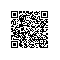# 03.Django的MTV开发模式详解和模型关系构建

ORM：对象关系映射

Django也遵循这种MVC开发模式，只不过更名为MTV，下边是django所对应的MVC
• M ，数据存取部分，由django数据库层处理，本章要讲述的内容。

• V ，选择显示哪些数据要显示以及怎样显示的部分，由视图和模板处理。

3
• C ，根据用户输入委派视图的部分，由 Django 框架根据 URLconf 设置，对给定 URL 调用适当的 Python 函数。

• M 代表模型（Model），即数据存取层。 该层处理与数据相关的所有事务： 如何存取、如何验证有效性、包含哪些行为以及数据之间的关系等。

• T 代表模板(Template)，即表现层。 该层处理与表现相关的决定： 如何在页面或其他类型文档中进行显示。

• V 代表视图（View），即业务逻辑层。 该层包含存取模型及调取恰当模板的相关逻辑。 你可以把它看作模型与模板之间的桥梁。

定义：注意先后顺序
（一个作者对应多本书）
class User(models.Model):    username = models.CharField(max_length=20)    password = models.CharField(max_length=20)def __unicode__(self):             #定义表内容显示的标题return self.usernameclass Meta:        #自定义数据表名称db_table = "User"

class Blog(models.Model):
#verbose_name='' : 表示后台中显示的对应名称
title = models.CharField(max_length=20, verbose_name='标题') #文章标题
author = models.ForeignKey(User,related_name="blog_author") #外键
#author = models.CharField(max_length=15,verbose_name='作者') #作者
time = models.TimeField(verbose_name='发表时间') #时间
tag = models.CharField(blank=True,max_length=100,verbose_name='文章标签') #文章标签 blank=True表示该空格可选填
content = models.TextField(verbose_name='内容') #内容

def __unicode__(self): #后台中显示的标题
return self.title

class Meta: #自定义数据表名字
db_table = "Blog"

注意先后顺序
（一个作者可以编写多种类别的文章，一个类别的文章可以被多个作者编写）
class Author(models.Model):    name = models.CharField(max_length=15)class Blog(models.Model):#verbose_name='' : 表示后台中显示的对应名称title = models.CharField(max_length=20, verbose_name='标题')   #文章标题authors = models.ManyToManyField(Author,verbose_name="作者")       #外键    #author = models.CharField(max_length=15,verbose_name='作者')  #作者time = models.TimeField(verbose_name='发表时间')      #时间tag = models.CharField(blank=True,max_length=100,verbose_name='文章标签')  #文章标签  blank=True表示该空格可选填content = models.TextField(verbose_name='内容')      #内容def __unicode__(self):   #后台中显示的标题return self.titleclass Meta:             #自定义数据表名字db_table = "Blog"class Category(models.Model):    category = models.CharField(max_length=20,verbose_name='类别')    blog = models.ForeignKey(Blog,related_name="category_blog")  #类别    author = models.ForeignKey(User,related_name="category_author")       #外键def __unicode__(self):return self.categoryclass Meta:         db_table = "Category"

class Author(models.Model):    name = models.CharField(max_length=15)
插入和更新数据a = Author(name='aaa')a.save()选择对象Author.objects.all()数据过滤Author.objects.filter(name='Apress',age=12) #以字典形式传值获取单个对象Author.objects.get(name='Apress')这样，就返回了单个对象，而不是列表（更准确的说，QuerySet)。 所以，如果结果是多个对象，会导致抛出异常如果查询没有返回结果也会抛出异常数据排序Author.order_by("name")连锁查询Author.objects.filter(name="sss").order_by("-name")限制返回的数据Author.order_by("name")更新多个对象Author.objects.filter(id=52).update(name='Apress Publishing')删除对象Author.objects.filter(name="sss").delete()

class Book(models.Model):  title = models.CharField(max_length=100)  genre = models.CharField(max_length=100)  num_pages = models.IntergerField()  authors = models.ManyToManyField(Author)def __unicode__(self):return self.titleclass Meta:    abstract = Trueclass SmithBook(Book):  authors = models.ManyToManyField(Author, limit_choices_to = {'name_endswith': 'Smith'  })

Meta类处理的是模型的各种元数据的使用和显示：

• 比如在一个对象对多个对象是，它的名字应该怎么显示；
• 查询数据表示默认的排序顺序是什么？
• 数据表的名字是什么
• 多变量唯一性 （这种限制没有办法在每个单独的变量声明上定义）
class Person(models.Model):
first = models.CharField(max_length=100)
last = models.CharField(max_length=100)
middle = models.CharField(max_length=100, blank=True)

class Meta:
ordering = ['last', 'first', 'middle']
unique_together = ['first', 'last', 'middle']

#Django默认的复数形式是加 s，这里不适用
verbose_name_plural = "people"使用钉钉扫一扫加入圈子
+ 订阅